﻿ MechDesigner Reference & User Interface > Dialog-Boxes > Dialog: Function-Block: Motion-Dimension

# Dialog: Function-Block: Motion-Dimension

Navigation:  MechDesigner Reference & User Interface > Dialog-Boxes >

# Dialog: Function-Block: Motion-Dimension

## Motion-Dimension FB

A Motion-Dimension FB

identifies a Part you want to move as a Motion-Part - a Rocker or Slider.

specifies the starting position of a Motion-Part

specifies the positive direction with increasing motion-values of a Motion-Part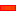Two FB icons in the Graphic-Area for a Motion-Dimension FB:

### Open Motion-Dimension dialog-box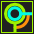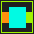Edit the Motion-Dimension FB that is in the model.

 1.Double-click the Motion-Dimension FB in the graphic-area or Assembly-Tree - or - 1.

The Motion-Dimension dialog-box is now open.

Motion-Dimension dialog-box

 The Motion-Dimension FB function is: Output = Base-Value + Input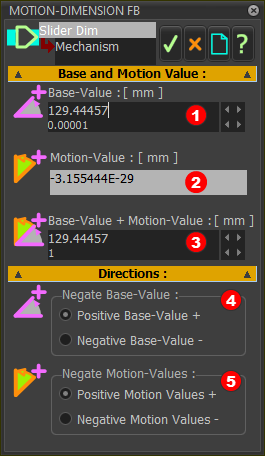Base and Motion ValueS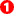Base-Value: The constant contribution to the output of the Motion-Dimension FB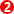Motion-Value: (read-only) The motion contribution to the output of the Motion-Dimension FB - equal to the motion-values at the input-connector.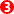Base-Value + Motion-Value (Constant + Motion) Base-Value + Motion-Value = Motion-Dimension at the Rocker or Slider.

Note: Units (angular or linear) are defined by the data-type at the input-connector.

Directions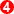Negate Motion-Values ◉ Positive Motion-Values : Motion-Value × 1 (default) ◉ Negative Motion-Values : Motion-Value × –1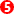Negate Base-Values ◉ Positive Base-Value : Base-Value × 1 ( default ) ◉ Negative Base-Value - Base-Value × –1

Notes:

Positive direction of a Motion-Dimension:

Rocker - counter-clockwise angle

Slider  - direction of arrowhead on Slide-Joint  -